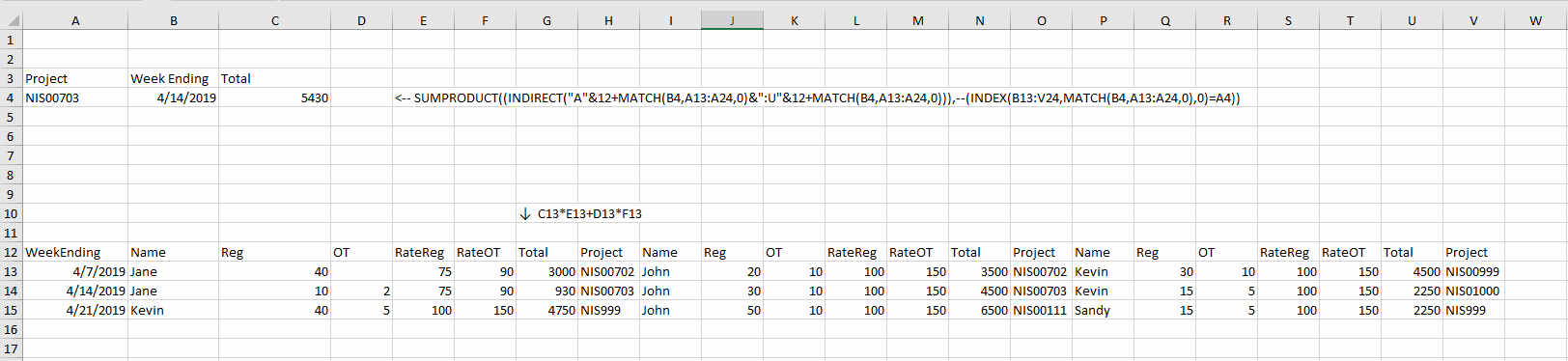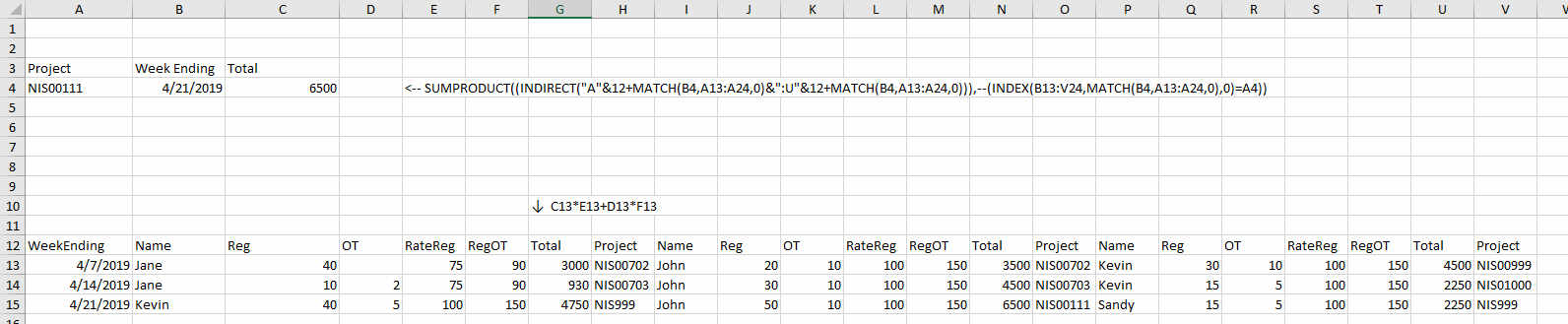Thread: Help with a formula needed please - sumifs formula not working - maybe another function needed?Thanks: 0Likes: 0

1.Help with a formula needed please - sumifs formula not working - maybe another function needed?

Good day. I'm setting up a worksheet that will track a contractor's daily labor, times it by their hourly rate, and provide a total cost for each week worked. That part works fine. The weekly costs are then associated with a project(or cost center). I have added an extra column to show which project(or cost center) the weekly costs are associated with.

After that, I'm having a problem. I want to write a formula that will look at each week, identify the project(or cost center) and sum the weekly amount for that project(or cost center).

The weekly total and the project number are on the same line.

Here is an example - Jane Doe worked 40 hours for the week ending 4/7/19. The total cost for the week is \$3,000, and the project ID is NIS00702. John Doe also worked 40 hours for the week ending 4/7/19, with total costs of \$3500, and the same project ID.

The formula should be able to find the project (NIS00702), and "sum" all costs that are associated with it for that week ending.

I tried a sumifs formula, but that is not working, because even though the total costs for the week are on the same line, they are not right next to each other (i.e., B13 - Regular Hours, C13 - OT Hours, D13 - Project ID, (Next Contractor) E13 - Regular hours, etc....

Any help in the right direction would be great! Thank you!Reply With Quote

2.Re: Help with a formula needed please - sumifs formula not working - maybe another function needed?

Hi SandyG - Hope this helps. A very simple example of SUMIFS has the formula =SUMIFS(A1:A3,B1:B3,"red") in Cell C1, with Cell A1 = 40, Cell A2 = 50, Cell A3 = 60, Cell B1 = red, Cell B2 = blue and Cell B3 = red. The SUMIFS looks for "red" in column B and then adds 40 + 60 to get 100. You should be able to do a similar thing in your example. Sometimes people forget to put quotes around the criteria (using red rather than "red") and that confuses the formula. Good luck.Reply With Quote

3.Re: Help with a formula needed please - sumifs formula not working - maybe another function needed?

SandyG - are all the contractors strung across ONE line based on the week ending? Where's the total cost for each?

Is it like this:

W/E Person RegHrs OTHrs RateReg RateOT Total Proj etc., etc.
4/7/19 Jane 40 0 75 100 3000 NIS00702 John 20 10 100 150 3500 NIS00702 ...

Or does the sheet have the W/E down the A column, person in B, etc., etc.?
If it's way, it's rather easy.

Column A is contractor name
Column B is HOURS
Column C is WeekEnding Date
Column D is Total
Column E is Proj Number

If G3 has the proj number to be found and H3 has the W/E date, I3 could be this formula:

Code:
=SUMIFS(D2:Dn,C2:Cn,H3,E2:En,G3)
You'd have to change the n to however many lines the listing is in.Reply With Quote

4.Re: Help with a formula needed please - sumifs formula not working - maybe another function needed?

If, in fact, your data is going horizontally, I think I have a solution to that format.
This version only tested w/three people but could be extended.Reply With Quote

User Tag List

Tags for this Thread

cost, hours, project, total, weekPosting Permissions

• You may not post new threads
• You may not post replies
• You may not post attachments
• You may not edit your posts
•